Latest Banking jobs   »

# Quantitative Aptitude Quiz For IBPS RRB PO/Clerk Mains 2022- 31st August

Q1. In a bag, there are 20 balls of three different color i.e. red, green and black. Ratio of number of red balls to that of green balls is 2: 3. If the probability of choosing a black ball from the bag is 0.25 then, find the probability of choosing a red and a green ball from the bag if the ball chosen is not replaced.
(a) 5/19
(b) 26/95
(c) 24/95
(d) 27/95
(e) None of these

Q2. A hemisphere is mounted on the base of cone and the radius of both is same i.e. 14 cm. Find the height of cone if the total volume of hemisphere and cone is 11,498 ⅔ cm³.
(a) 35 cm
(b) 21 cm
(c) 31 ½ cm
(d) 28 cm
(e) 24.5 cm

Q3. 60 men together complete a work in 15 days while 18 women together complete that work in 40 days. All women started working together and on every next day 6 women left the work and after three days, 25 men joined to do the remaining work then find in how many days work will be completed?
(a) 33 ⅕ days
(b) 34 ⅕ days
(c) 35 days
(d) 33 ½ days
(e) 32 ½ days

Q4. A shopkeeper allows two successive discounts of 15% and 10% on marked price of an article and customer also managed to get additional 10% discount on discounted price and in this way, he gets an additional discount of Rs.459. Find the price at which customer bought the article?
(a) Rs. 4193
(b) Rs. 4281
(c) Rs. 4277
(d) Rs. 4131
(e) Rs. 4099

Q5. Amount invested by C is Rs 4000 more than that of A and after 9 months, A and C both withdrew their whole amount and B joined the business with Rs. 24,000. If at the end of year, ratio of profit share of A to that of C is 2:3 then, find profit share by B out of total profit of Rs 14000?
(a) Rs. 3600
(b) Rs. 4000
(c) Rs. 4400
(d) Rs. 3200
(e) Rs. 4000

Q6. Train A travelling at the speed of 135 kmph overtook train B, which travelling in the same direction in 12 sec. The length of train A is two times of train B. If train B travels at twice of its speed, then train A would have taken 24 sec, then find the length of train B.
(a) 200 meter
(b) 150 meter
(c) 75 meter
(d) 100 meter
(e) 135 meter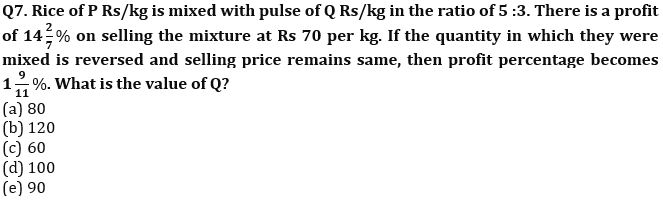Q8. Dharmender and Shivam invested Rs 24000 and Rs 20000 respectively in a business. After 8 months of investment Shivam invested Rs 4000 more while Dharmender invested Rs 6000 more. At the end of the year the profit was Rs 149100. If Dharmender invested his profit share on Simple interest at 16⅔% for 2 years and Shivam invested his profit share on compound interest at 12.5% for two years, then find the difference between the interest earned by them.
(a) Rs 4590
(b) Rs 8460
(c) Rs 7850
(d) Rs 9450
(e) Rs 10500

Q9. 2k years ago, the ratio of age of A and B is 6 :11. The ratio of their ages was 3:7, 5k years ago. If 3k years later, the sum of their ages will be 98 years, then find the difference between their present ages.
(a) 40 years
(b) 25 years
(c) 80 years
(d) 70 years
(e) 20 years

Q10. Two friends Sanjay and Praveen bought two cars. Sanjay bought from store P where he get 25% discount and Praveen bought from store Q where he get two successive discount of d% and 10%. The market price of both cars on both stores is same. Sanjay bought the car at Rs 2,40,000. If Praveen paid Rs 4800 more than Sanjay, find the discount ‘d’?
(a) 18%
(b) 20%
(c) 25%
(d) 15%
(e) 12.5%

Q11. A,B,C and D working alone can complete a work in 5,6,7 and 8 days respectively. At the most only one person can work in each day and no one can work for two consecutive days. Find the minimum number of days required to complete the work?
(a) 46⅔
(b) 5⅖
(c) 7⅖
(d) 9⅖
(e)11⅖

Q12. A vessel of capacity 144 liters contains three types of volatile liquid A,B,C in the ratio of 1:2:3.The liquid in the vessel evaporates in the ratio of 3:2:1 when kept in open. Find the total amount of liquid A and liquid C left in the vessel if 12 L of liquid B got evaporated? (in liters)
(a)62
(b)24
(c)40
(d)66
(e)72

Q13. Cost price of an article is 39% less than the marked price of the article and shopkeeper earned 40% profit in selling the article. If amount of profit is Rs.196 more than amount of discount, then find cost price of article.
(a) Rs.1345
(b) Rs.1325
(c) Rs.1290
(d) Rs.1245
(e) Rs.1220

Q14. Time taken by boat to cover 48 km in upstream is 200% of the time taken by boat to cover 48 km in downstream. If sum of time taken by boat to cover 48 km distance in upstream and same distance in downstream is 9 hours, then find speed of boat?
(a) 8 km/hr
(b) 10 km/hr
(c) 12 km/hr
(d) 4 km/hr
(e) 6 km/hr

Q15. Pankaj and Suresh invested capital in the ratio of 4 : 3 in a partnership business. Pankaj and Suresh left the business after 5 months and after 10 months respectively. Raju joined the business with Rs.50000 when Suresh left, and they wind up the business after 1 year. If at the end of one-year profit sharing ratio of Pankaj, Suresh & Raju is 2 : 3 : 1, then find the difference between the capital invested by Pankaj and Suresh?
(a) Rs.10000
(b) Rs.40000
(c) Rs.30000
(d) Rs.50000
(e) Rs.20000

Solutions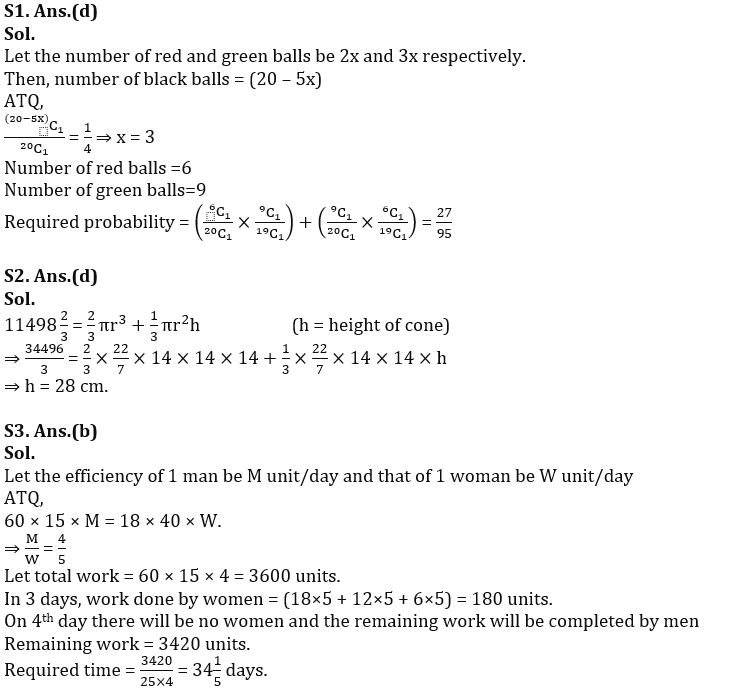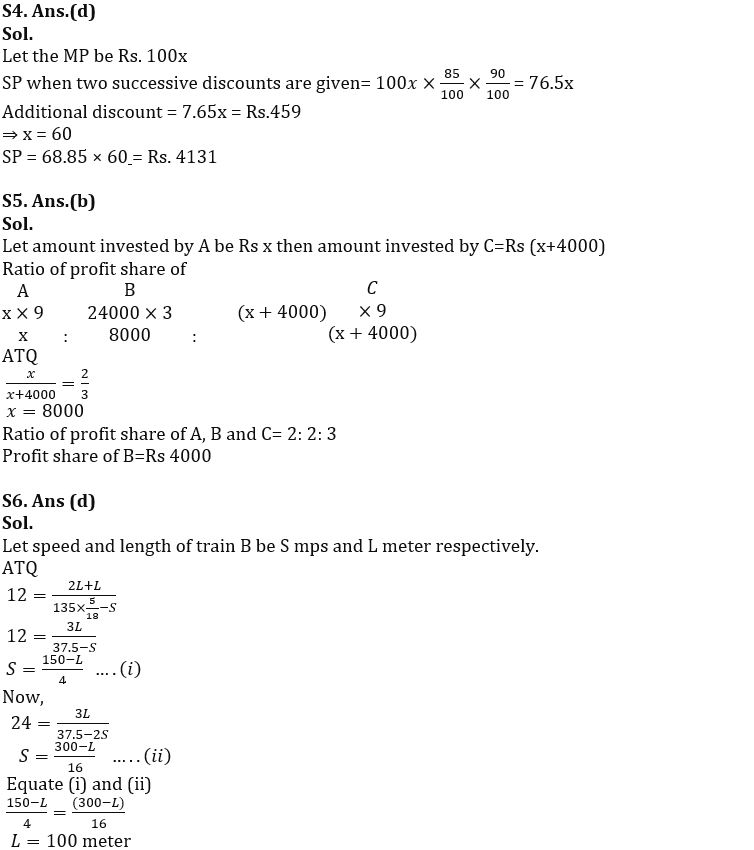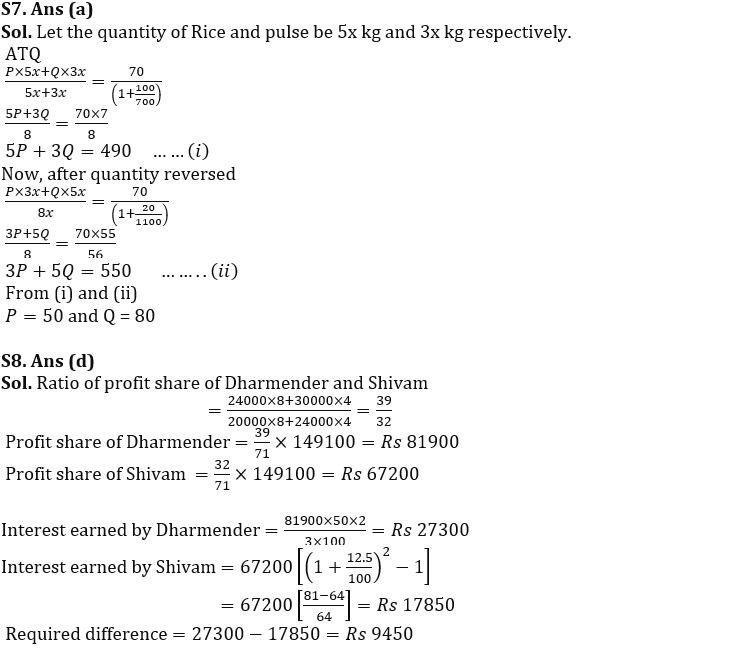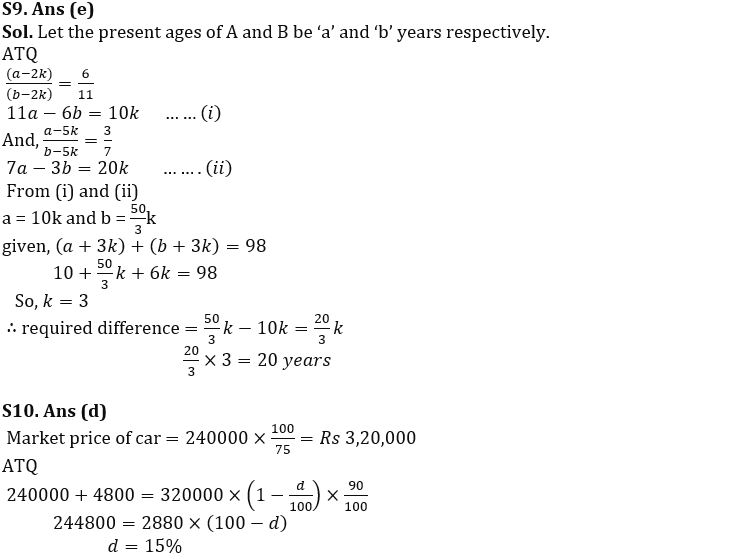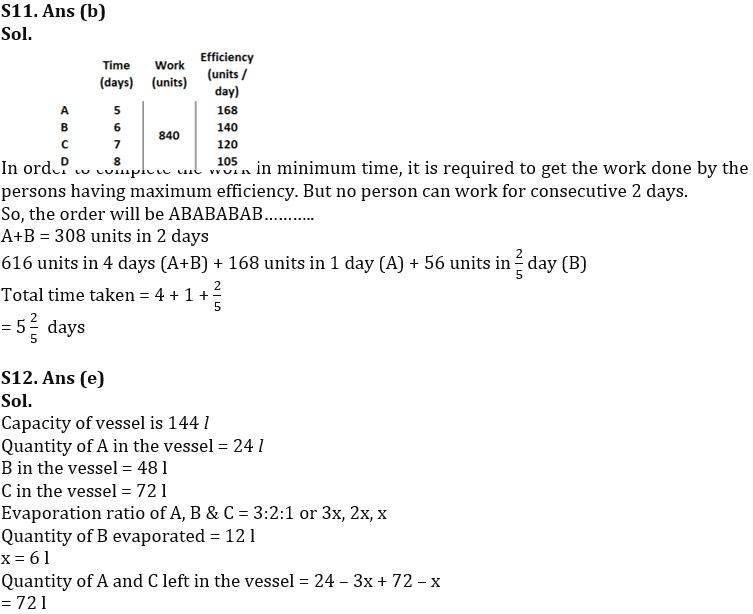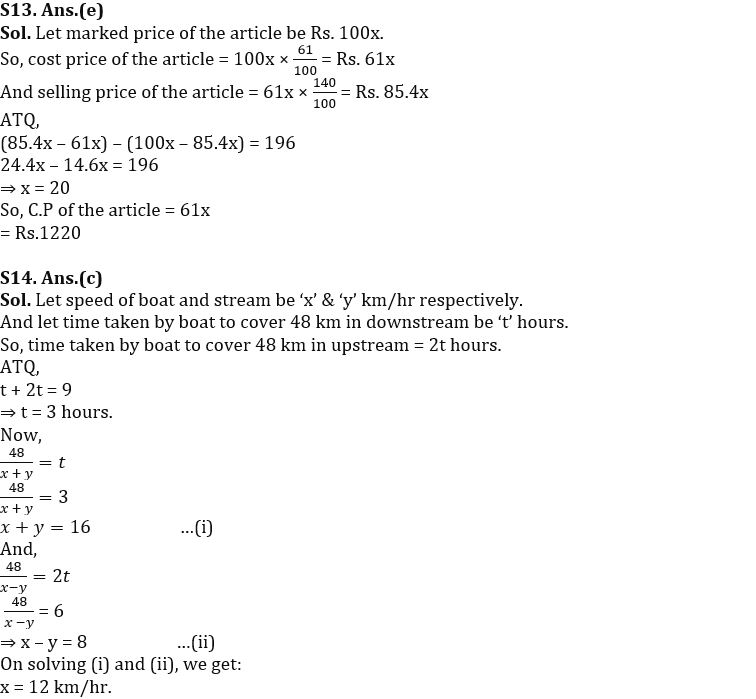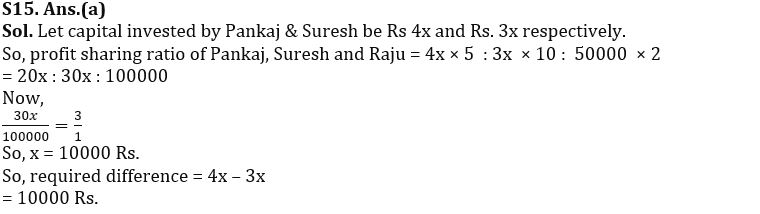#### Congratulations!Download Hindu Review of October 2021: Free PDF